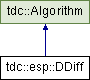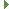tudocomp – The TU Dortmund Compression Framework
tdc::esp::DDiff Class Reference

#include <DRCoder.hpp>

Inheritance diagram for tdc::esp::DDiff:Public Member Functions

template<typename rhs_t >
void encode (const rhs_t &rhs, BitOStream &out, size_t bit_width, size_t max_value) const

template<typename rhs_t >
void decode (rhs_t &rhs, BitIStream &in, size_t bit_width, size_t max_value) const

template<typename rhs_t >
void encode (const rhs_t &rhs, std::shared_ptr< BitOStream > &out, size_t bit_width, size_t max_value) const

template<typename rhs_t >
void decode (rhs_t &rhs, std::shared_ptr< BitIStream > &in, size_t bit_width, size_t max_value) constPublic Member Functions inherited from tdc::Algorithm
virtual ~Algorithm ()=default

Algorithm (Algorithm const &)=default

Algorithm (Algorithm &&)=default

Algorithmoperator= (Algorithm const &)=default

Algorithmoperator= (Algorithm &&)=default

Algorithm (Env &&env)
Instantiates an algorithm in the specified environment. More...

Envenv ()

const Envenv () const

Static Public Member Functions

static Meta meta ()

Detailed Description

Definition at line 485 of file DRCoder.hpp.

◆ decode() [1/2]

template<typename rhs_t >
 void tdc::esp::DDiff::decode ( rhs_t & rhs, BitIStream & in, size_t bit_width, size_t max_value ) const
inline

Definition at line 500 of file DRCoder.hpp.

◆ decode() [2/2]

template<typename rhs_t >
 void tdc::esp::DDiff::decode ( rhs_t & rhs, std::shared_ptr< BitIStream > & in, size_t bit_width, size_t max_value ) const
inline

Definition at line 508 of file DRCoder.hpp.

◆ encode() [1/2]

template<typename rhs_t >
 void tdc::esp::DDiff::encode ( const rhs_t & rhs, BitOStream & out, size_t bit_width, size_t max_value ) const
inline

Definition at line 495 of file DRCoder.hpp.

◆ encode() [2/2]

template<typename rhs_t >
 void tdc::esp::DDiff::encode ( const rhs_t & rhs, std::shared_ptr< BitOStream > & out, size_t bit_width, size_t max_value ) const
inline

Definition at line 504 of file DRCoder.hpp.

◆ meta()

 static Meta tdc::esp::DDiff::meta ( )
inlinestatic

Definition at line 487 of file DRCoder.hpp.

The documentation for this class was generated from the following file: